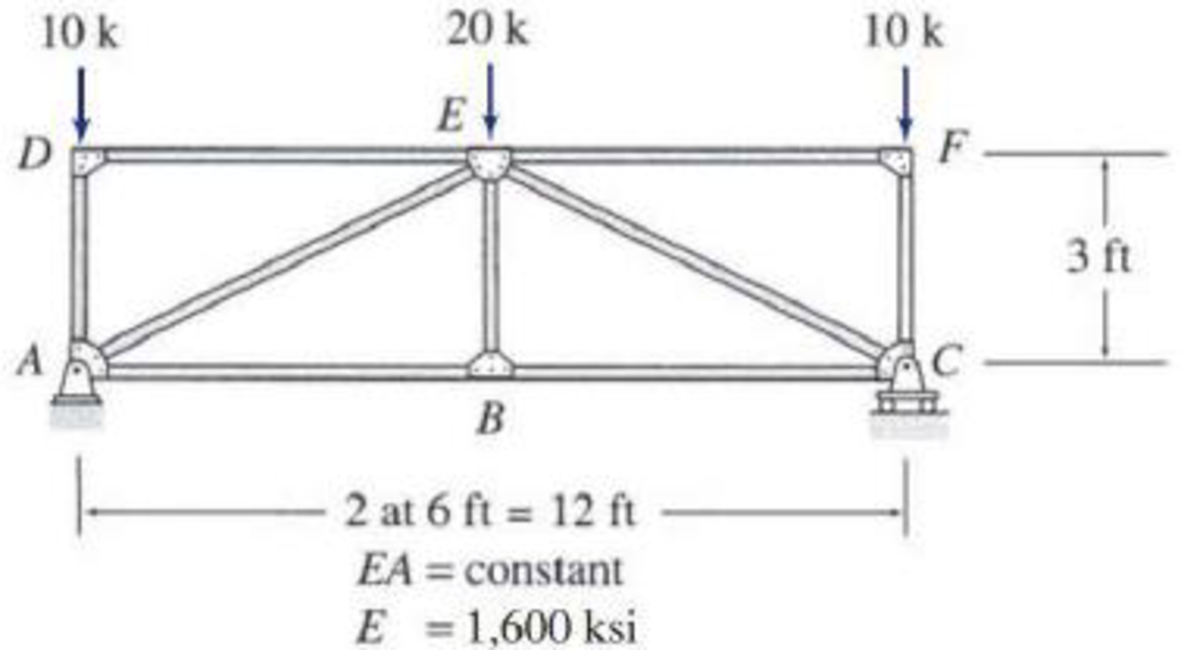# 7.13 through 7.15 Determine the smallest cross-sectional area A for the members of the truss shown, so that the vertical deflection at joint B does not exceed 0.4 inches. Use the method of virtual work.

#### Solutions

Chapter
Section
Chapter 7, Problem 14P
Textbook Problem
80 views

## 7.13 through 7.15 Determine the smallest cross-sectional area A for the members of the truss shown, so that the vertical deflection at joint B does not exceed 0.4 inches. Use the method of virtual work.To determine

Calculate the smallest cross sectional area of the truss.

### Explanation of Solution

Given information:

The truss is given in the Figure.

The vertical deflection at joint B is 0.4 in.

The value of E is 1,600 ksi.

Procedure to find the deflection of truss by virtual work method is shown below.

• For real system: If the deflection of truss is determined by the external loads, then apply method of joints or method of sections to find the real axial forces (F) in all the members of the truss.
• For virtual system: Remove all given real loads, apply a unit load at the joint where is deflection is required and also in the direction of desired deflection. Use method of joints or method of sections to find the virtual axial forces (Fv) in all the member of the truss.
• Finally use the desired deflection equation.

Apply the sign conventions for calculating reactions, forces and moments using the three equations of equilibrium as shown below.

• For summation of forces along x-direction is equal to zero (Fx=0), consider the forces acting towards right side as positive (+) and the forces acting towards left side as negative ().
• For summation of forces along y-direction is equal to zero (Fy=0), consider the upward force as positive (+) and the downward force as negative ().
• For summation of moment about a point is equal to zero (Matapoint=0), consider the clockwise moment as negative and the counter clockwise moment as positive.

Method of joints:

The negative value of force in any member indicates compression (C) and the positive value of force in any member indicates tension (T).

Condition for zero force members:

1. 1. If only two non-collinear members are connected to a joint that has no external loads or reactions applied to it, then the force in both the members is zero.
2. 2. If three members, two of which are collinear are connected to a joint that has no external loads or reactions applied to it, then the force in non-collinear member is zero.

Calculation:

Consider the real system.

Find the member axial force (F) for the real system using method of joints:

Let Ax and Ay be the horizontal and vertical reactions at the hinged support A.

Let By be the vertical reaction at the roller support B.

Sketch the resultant diagram of the truss as shown in Figure 1.

Find the reactions at the supports using equilibrium equations:

Summation of moments about A is equal to 0.

MA=0By(12)10(12)20(6)=0By=20k

Summation of forces along y-direction is equal to 0.

+Fy=0Ay+By102010=0Ay+20102010=0Ay=20k

Summation of forces along x-direction is equal to 0.

+Fx=0Ax=0

Find the member forces using method of joints:

Apply equilibrium equation to the joint D:

Summation of forces along y-direction is equal to 0.

Summation of forces along x-direction is equal to 0.

+Fx=0FDE=0

Apply equilibrium equation to the joint F:

Summation of forces along x-direction is equal to 0.

+Fx=0FEF=0

Summation of forces along y-direction is equal to 0.

+Fy=010FCF=0FCF=10k

Apply equilibrium equation to the joint A:

Summation of forces along y-direction is equal to 0.

Summation of forces along x-direction is equal to 0.

+Fx=0FAB+FAEcos26.565°=0FAB+(22.36)cos26.565°=0FAB=20k

Apply equilibrium equation to the joint C:

Summation of forces along y-direction is equal to 0.

+Fy=020+FCF+FCEsin26.565°=020+(10)+FCEsin26.565°=0FCE=22.36k

Summation of forces along x-direction is equal to 0.

+Fx=0FBCFCEcos26.565°=0FBC(22.36)cos26.565°=0FBC=20k

Apply equilibrium equation to the joint E:

Summation of forces along y-direction is equal to 0.

+Fy=020FBEFAEsin26.565°FCEsin26.565°=020FBE(22.36)sin26.565°(22.36)sin26.565°=0FBE=0

Consider the virtual system:

For vertical deflection apply l kN at joint B in the vertical direction.

Find the member axial force (Fv) due to virtual vertical load using method of joints:

Sketch the resultant diagram of the virtual system (Fv) as shown in Figure 2.

Find the reactions at the supports using equilibrium equations:

Summation of moments about A is equal to 0.

MA=0By(12)1(6)=0By=0.5k

Summation of forces along y-direction is equal to 0

### Still sussing out bartleby?

Check out a sample textbook solution.

See a sample solution

#### The Solution to Your Study Problems

Bartleby provides explanations to thousands of textbook problems written by our experts, many with advanced degrees!

Get Started

Find more solutions based on key concepts
Determine the pressure required to decrease the volume of the following fluids by 2%: a. water b. glycerin c. S...

Engineering Fundamentals: An Introduction to Engineering (MindTap Course List)

Define the term, optical disc. List types of optical discs.

Enhanced Discovering Computers 2017 (Shelly Cashman Series) (MindTap Course List)

6. How would you level DFDs?

Systems Analysis and Design (Shelly Cashman Series) (MindTap Course List)

What element is in stainless steel that makes it corrosion resistant?

Precision Machining Technology (MindTap Course List)

If your motherboard supports ECC DDR3 memory, can you substitute non-ECC DDR3 memory?

A+ Guide to Hardware (Standalone Book) (MindTap Course List)